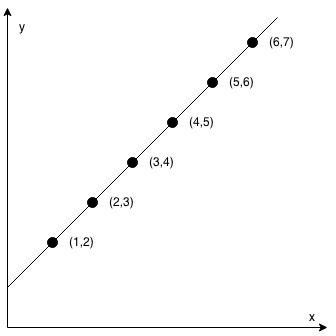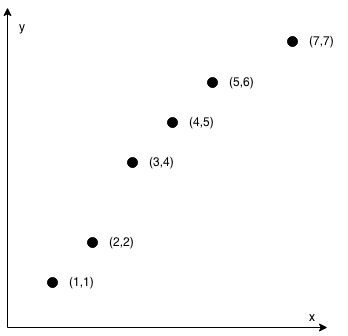Leetcode 1232：缀点成线（超详细的解法！！！）
in leetcode with 0 comment输入：coordinates = [[1,2],[2,3],[3,4],[4,5],[5,6],[6,7]]输入：coordinates = [[1,1],[2,2],[3,4],[4,5],[5,6],[7,7]]

• 2 <= coordinates.length <= 1000
• coordinates[i].length == 2
• -10^4 <= coordinates[i], coordinates[i] <= 10^4
• coordinates 中不含重复的点

• $y_1=ax_1+b$
• $y_2=ax_2+b$

• $a=\frac{y_1-y_2}{x_1-x_2}$
• $b=y_1-ax_1$

• $\frac{y_1-y_3}{x_1-x_3}=\frac{y_2-y_3}{x_2-x_3}$

• $(y_1-y_3)*(x_2-x_3)=(y_2-y_3)*(x_1-x_3)$
class Solution:
def checkStraightLine(self, c: List[List[int]]) -> bool:
return all((x - c) * (y - c) == (x - c) * (y - c) for x, y in c[2:])

reference:

https://leetcode.com/problems/check-if-it-is-a-straight-line/discuss/408984/JavaPython-3-check-slopes-short-code-w-explanation-and-analysis.

「如果我的文章对你有很大帮助，那么不妨～！」Responses